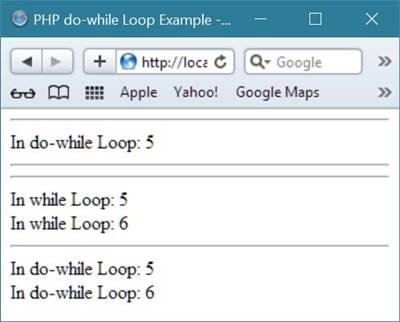# PHP do-while Loop

PHP do-while loop is used to execute a block of code at least once.

The do-while loop in PHP, is similar to the PHP while loop, but in case of do-while loop, the condition-checker-expression is evaluated at the bottom of the loop. Here is the general form of the do-while loop in PHP:

```do
{
code to be executed here;
}while (condition);```

## PHP do-while Loop Example

Here is an example used do-while loop in PHP. This example will decrement the value of the variable i at least once, and it will continue decrementing the value of the variable i until its value become equal to 0

```<html>
<title>PHP do-while Loop Example - CodesCracker</title>
<body>
<?php
\$i = 25;
do
{
echo \$i." ";
\$i--;
}while(\$i>0);
echo "Fire!!!<br/>";
echo "Loop stopped at i = ".\$i;
?>
</body>
</html>```

Here is the output of the above PHP script:Let's take another example also demonstrates do-while loop in PHP.

```<html>
<title>PHP do-while Loop Example - CodesCracker</title>
<body>
<?php
\$number = 2;
\$i = 1;
do
{
echo \$number * \$i;
echo "<br/>";
\$i++;
}while(\$i<=10);
?>
</body>
</html>```

The sample output produced by the above do-while loop example code is shown here:Let's take last example on do-while loop in PHP.

```<html>
<title>PHP do-while Loop Example - CodesCracker</title>
<body>
<?php
\$numb = 5;
while(\$numb<5)
{
echo "In while Loop: ".\$numb."<br/>";
\$numb++;
}
echo "<hr/>";
\$numb = 5;
do
{
echo "In do-while Loop: ".\$numb."<br/>";
\$numb++;
}while(\$numb<5);

echo "<hr/><hr/>";

\$numb = 5;
while(\$numb<7)
{
echo "In while Loop: ".\$numb."<br/>";
\$numb++;
}
echo "<hr/>";
\$numb = 5;
do
{
echo "In do-while Loop: ".\$numb."<br/>";
\$numb++;
}while(\$numb<7);
?>
</body>
</html>```

Below is the sample output of the above do-while example.## Difference between while and do-while Loop

The PHP while loop first evaluates its condition then enters into its block if the condition evaluates to be true, whereas in case of do-while loop, its condition evaluates at last of the loop, and before evaluating the condition, the content inside the do-while will be executed at the first time without mannering about the condition whether it will be true or false, and after executing the block of code inside the do-while loop, the program control then goes to the condition part to check if the condition evaluates to be true or false, if it is true, then program control again enters into the block of the loop.

In simple line, while loop evaluates its condition then enters into the block, whereas do-while loop enters into the block then evaluates its condition.

Or you can say that,

• for while loop - check before execute
• for do-while loop - execute before check (for first time only)

Tools
Calculator